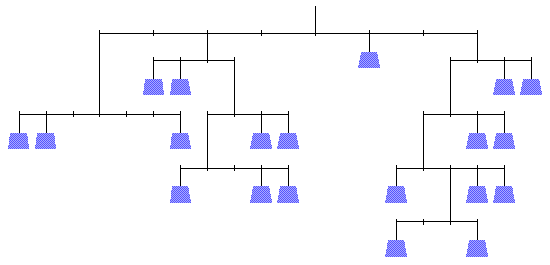Weight Puzzles

The weights in the following puzzles have whole number weights 1, 2, 3, . . . up to n, where n is the number of weights. In each case, the horizontal rods indicate there is equal torque on both sides. (The torque of each weight is its weight times its distance from the fulcrum.) Assume the strings and rods have negligible weight. The example below balances since 2(1) + 1(2) = 1(4) and 3(3) + 1(5) = 2(7).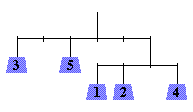Each puzzle below has a unique solution.

 5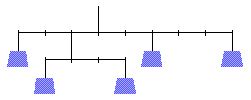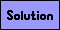6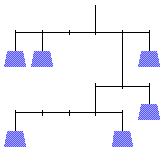7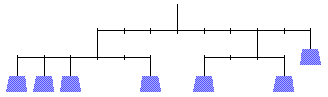8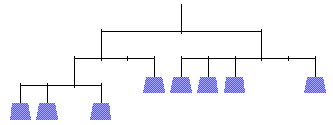9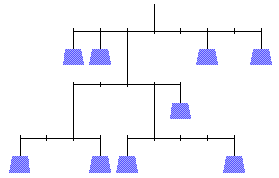10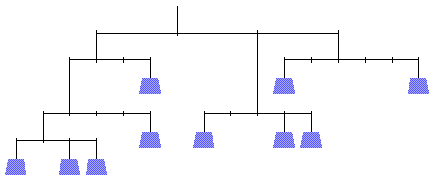11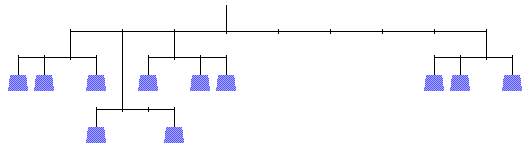12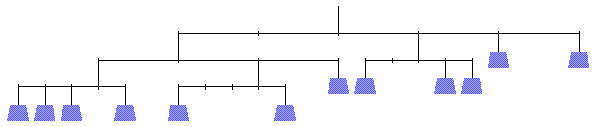13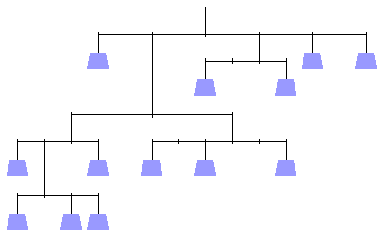14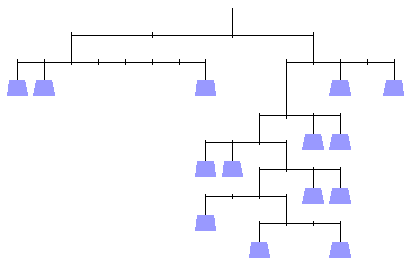15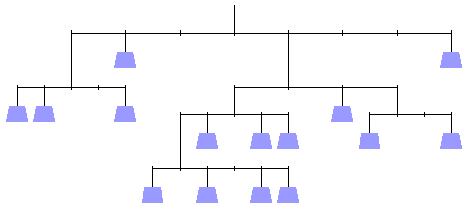16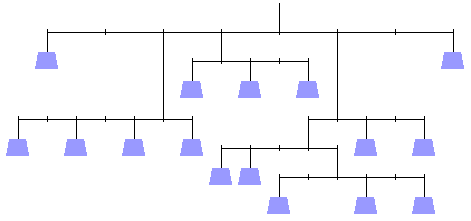17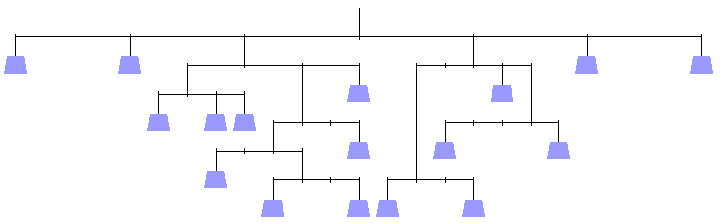18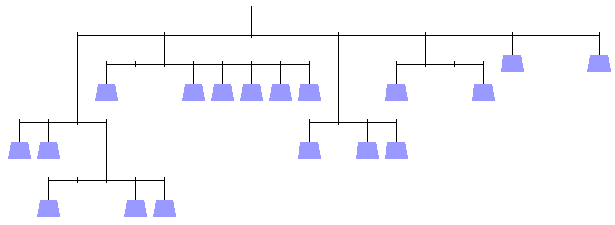19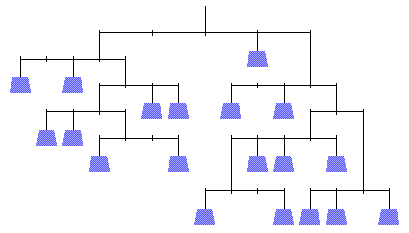20